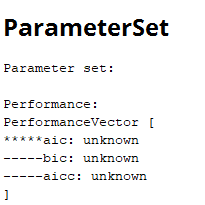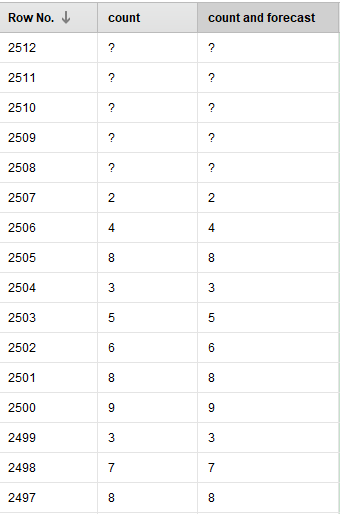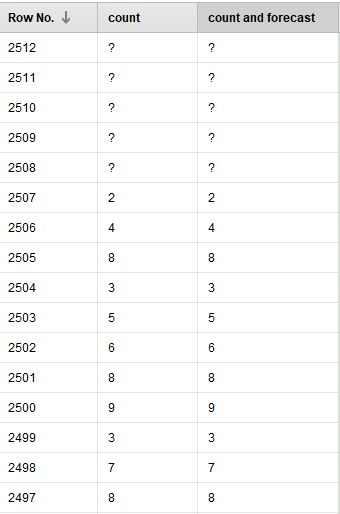# ARIMA loop model get stucked always and do not complete the execution

Member Posts: 27Contributor I
edited December 2018 in Help

Hello everyone,

I've created a process to make forcast on a time series using arima(p,d,q) model inside a loop that changes p,d,q parameters(Optimize Parameters (Grid)) within a certain range. the problem is that once I enlarge the range of each parameter the execution don't provide forecast output anymore, which is really odd.???

Here is an example proving that the execution don't provide results:ANDI do really need your help guys.

Best regards.

Tagged:

• Administrator, Employee, RapidMiner Certified Analyst, RapidMiner Certified Expert, RMResearcher, Member Posts: 164RM Research

Hi @data1maths,

Would it be possible to provide your process (as xml) and best a sample dataset (or a one which is as similar as possible), to investigate in more detail what is happening?

Best regards,

Fabian

• Member Posts: 27Contributor I

Hi

Here is the process(xml).

Thank you very much.

Best regards

`<?xml version="1.0" encoding="UTF-8"?><process version="9.0.003">  <context>    <input/>    <output/>    <macros/>  </context>  <operator activated="true" class="process" compatibility="9.0.003" expanded="true" name="Process">    <process expanded="true">      <operator activated="true" class="retrieve" compatibility="9.0.003" expanded="true" height="68" name="Retrieve Time series-mvts de congestion" width="90" x="112" y="136">        <parameter key="repository_entry" value="Time series-mvts de congestion"/>      </operator>      <operator activated="true" class="set_macros" compatibility="9.0.003" expanded="true" height="82" name="Set Macros" width="90" x="380" y="34">        <list key="macros">          <parameter key="dateTime" value="'PILOT ON'"/>        </list>      </operator>      <operator activated="true" class="generate_attributes" compatibility="9.0.003" expanded="true" height="82" name="Generate Attributes" width="90" x="313" y="289">        <list key="function_descriptions">          <parameter key="count" value="1"/>        </list>      </operator>      <operator activated="true" class="select_attributes" compatibility="9.0.003" expanded="true" height="82" name="Select Attributes" width="90" x="447" y="289">        <parameter key="attribute_filter_type" value="subset"/>        <parameter key="attributes" value="count|PILOT ON"/>      </operator>      <operator activated="true" class="generate_id" compatibility="9.0.003" expanded="true" height="82" name="Generate ID" width="90" x="514" y="187"/>      <operator activated="true" class="python_scripting:execute_python" compatibility="8.2.000" expanded="true" height="103" name="Execute Python" width="90" x="715" y="34">        <parameter key="script" value="import pandas as pd&#10;&#10;# rm_main is a mandatory function, &#10;# the number of arguments has to be the number of input ports (can be none)&#10;DT = %{dateTime}&#10;&#10;def rm_main(data):&#10;&#10;  data[DT] = pd.to_datetime(data[DT])&#10;  data = data.set_index(DT)&#10;  data = data.resample('4H', how='count')&#10;  data = data.reset_index()&#10;    &#10;  #data.rm_metadata[&quot;Datetime&quot;] = (None,&quot;regular&quot;)&#10;    &#10;    # connect 2 output ports to see the results&#10;  return data"/>      </operator>      <operator activated="false" class="multiply" compatibility="9.0.003" expanded="true" height="82" name="Multiply" width="90" x="782" y="136"/>      <operator activated="false" class="write_excel" compatibility="9.0.003" expanded="true" height="82" name="Write Excel" width="90" x="849" y="289">        <parameter key="excel_file" value="C:\Users\HP\Desktop\tmpa\Mental strenght\dataset sample-RM-cong.xlsx"/>      </operator>      <operator activated="true" breakpoints="after" class="concurrency:optimize_parameters_grid" compatibility="9.0.003" expanded="true" height="166" name="Optimize Parameters (Grid)" origin="GENERATED_SAMPLE" width="90" x="916" y="34">        <list key="parameters">          <parameter key="ARIMA Original Data.plithiumorder_of_the_autoregressive_model" value="[1;24;24;linear]"/>          <parameter key="ARIMA Original Data.dlithiumdegree_of_differencing" value="[0.0;2;2;linear]"/>          <parameter key="ARIMA Original Data.qlithiumorder_of_the_moving-average_model" value="[0;24;24;linear]"/>        </list>        <process expanded="true">          <operator activated="true" class="time_series:arima_trainer" compatibility="9.0.003" expanded="true" height="103" name="ARIMA Original Data" origin="GENERATED_SAMPLE" width="90" x="380" y="34">            <parameter key="time_series_attribute" value="count"/>            <parameter key="indices_attribute" value="id"/>            <parameter key="plithiumorder_of_the_autoregressive_model" value="24"/>            <parameter key="dlithiumdegree_of_differencing" value="2"/>            <parameter key="qlithiumorder_of_the_moving-average_model" value="24"/>          </operator>          <connect from_port="input 1" to_op="ARIMA Original Data" to_port="example set"/>          <connect from_op="ARIMA Original Data" from_port="forecast model" to_port="output 1"/>          <connect from_op="ARIMA Original Data" from_port="performance" to_port="performance"/>          <connect from_op="ARIMA Original Data" from_port="original" to_port="output 2"/>          <portSpacing port="source_input 1" spacing="0"/>          <portSpacing port="source_input 2" spacing="0"/>          <portSpacing port="sink_performance" spacing="0"/>          <portSpacing port="sink_model" spacing="0"/>          <portSpacing port="sink_output 1" spacing="0"/>          <portSpacing port="sink_output 2" spacing="0"/>          <portSpacing port="sink_output 3" spacing="0"/>        </process>      </operator>      <operator activated="true" class="time_series:apply_forecast" compatibility="9.0.003" expanded="true" height="82" name="Apply Forecast" origin="GENERATED_SAMPLE" width="90" x="1050" y="187"/>      <connect from_op="Retrieve Time series-mvts de congestion" from_port="output" to_op="Set Macros" to_port="through 1"/>      <connect from_op="Set Macros" from_port="through 1" to_op="Generate Attributes" to_port="example set input"/>      <connect from_op="Generate Attributes" from_port="example set output" to_op="Select Attributes" to_port="example set input"/>      <connect from_op="Select Attributes" from_port="example set output" to_op="Generate ID" to_port="example set input"/>      <connect from_op="Generate ID" from_port="example set output" to_op="Execute Python" to_port="input 1"/>      <connect from_op="Execute Python" from_port="output 1" to_op="Optimize Parameters (Grid)" to_port="input 1"/>      <connect from_op="Multiply" from_port="output 1" to_op="Write Excel" to_port="input"/>      <connect from_op="Optimize Parameters (Grid)" from_port="performance" to_port="result 3"/>      <connect from_op="Optimize Parameters (Grid)" from_port="model" to_port="result 4"/>      <connect from_op="Optimize Parameters (Grid)" from_port="parameter set" to_port="result 5"/>      <connect from_op="Optimize Parameters (Grid)" from_port="output 1" to_op="Apply Forecast" to_port="forecast model"/>      <connect from_op="Apply Forecast" from_port="example set" to_port="result 1"/>      <connect from_op="Apply Forecast" from_port="original" to_port="result 2"/>      <portSpacing port="source_input 1" spacing="0"/>      <portSpacing port="sink_result 1" spacing="0"/>      <portSpacing port="sink_result 2" spacing="0"/>      <portSpacing port="sink_result 3" spacing="0"/>      <portSpacing port="sink_result 4" spacing="0"/>      <portSpacing port="sink_result 5" spacing="0"/>      <portSpacing port="sink_result 6" spacing="0"/>      <portSpacing port="sink_result 7" spacing="0"/>    </process>  </operator></process>`